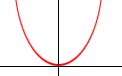Quandaries and Queries Name: Audrey Who is asking: Student Level: Secondary Question: How do you estimate the equation of a parabola? I have a project where a must find parabolas in magazines and then estimate their equation.HELP Hi Audrey, When you find a parabola in a magazine, turn the page so that the parabola opens upward. Draw X and Y axes on the page so that the vertex of the parabola is at the origin and positive Y-axis is upwards.In this position the parabola has equation y = k x 2 for some number k. Notice that when x = 1, y = k. Using whatever units you are going to use, find the point (1,0) on the X-axis. The point on the parabola directly above this point has coordinates (1,k). Mearure the distance from the X-axis to this point to find k. Penny Go to Math Central